The VARMAX Procedure

VAR and VARX Modeling

Subsections:

The pth-order VAR process is written as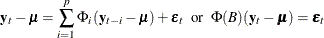with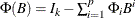.

Equivalently, it can be written as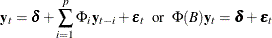with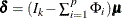.

Stationarity

For stationarity, the VAR process must be expressible in the convergent causal infinite MA form as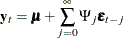where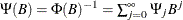with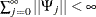, where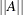denotes a norm for the matrix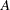such as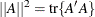. The matrix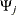can be recursively obtained from the relation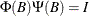; it is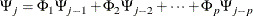where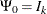and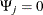for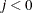.

The stationarity condition is satisfied if all roots of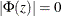are outside of the unit circle. The stationarity condition is equivalent to the condition in the corresponding VAR(1) representation,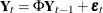, that all eigenvalues of the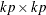companion matrix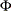be less than one in absolute value, where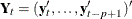,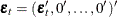, and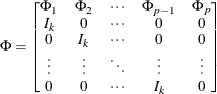If the stationarity condition is not satisfied, a nonstationary model (a differenced model or an error correction model) might be more appropriate.

The following statements estimate a VAR(1) model and use the ROOTS option to compute the characteristic polynomial roots:

proc varmax data=simul1;
model y1 y2 / p=1 noint print=(roots);
run;


Figure 35.44 shows the output associated with the ROOTS option, which indicates that the series is stationary since the modulus of the eigenvalue is less than one.

Figure 35.44: Stationarity (ROOTS Option)

The VARMAX Procedure

Roots of AR Characteristic Polynomial
Index Real Imaginary Modulus Radian Degree
1 0.77238 0.35899 0.8517 0.4351 24.9284
2 0.77238 -0.35899 0.8517 -0.4351 -24.9284

Parameter Estimation

Consider the stationary VAR(p) model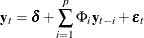where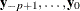are assumed to be available (for convenience of notation). This can be represented by the general form of the multivariate linear model,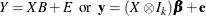where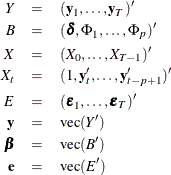with vec denoting the column stacking operator.

The conditional least squares estimator ofis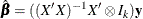and the estimate of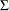is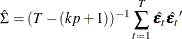where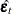is the residual vectors. Consistency and asymptotic normality of the LS estimator are that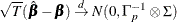where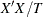converges in probability to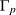and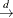denotes convergence in distribution.

The (conditional) maximum likelihood estimator in the VAR(p) model is equal to the (conditional) least squares estimator on the assumption of normality of the error vectors.

Asymptotic Distributions of Impulse Response Functions

As before, vec denotes the column stacking operator and vech is the corresponding operator that stacks the elements on and below the diagonal. For any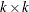matrix, the commutation matrix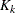is defined as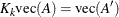; the duplication matrix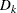is defined as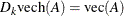; the elimination matrix
is defined as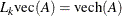.

The asymptotic distribution of the impulse response function (Lütkepohl, 1993) is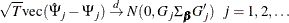where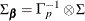and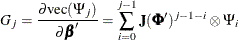where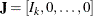is a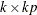matrix and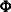is a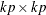companion matrix.

The asymptotic distribution of the accumulated impulse response function is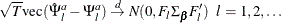where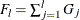.

The asymptotic distribution of the orthogonalized impulse response function is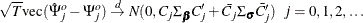where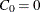,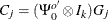,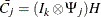,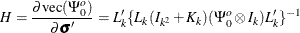and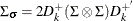with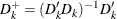and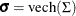.

Granger Causality Test

Let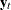be arranged and partitioned in subgroupsand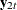with dimensions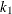and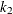, respectively (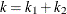); that is,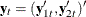with the corresponding white noise process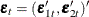. Consider the VAR(p) model with partitioned coefficients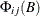for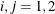as follows: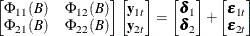The variablesare said to cause, butdo not causeif. The implication of this model structure is that future values of the processare influenced only by its own past and not by the past of, where future values ofare influenced by the past of bothand. If the futureare not influenced by the past values of, then it can be better to modelseparately from.

Consider testing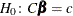, where C is a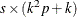matrix of rank s and c is an s-dimensional vector where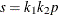. Assuming thatyou get the Wald statistic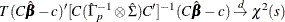For the Granger causality test, the matrix C consists of zeros or ones and c is the zero vector. See Lütkepohl (1993) for more details of the Granger causality test.

VARX Modeling

The vector autoregressive model with exogenous variables is called the VARX(p,s) model. The form of the VARX(p,s) model can be written as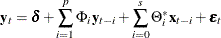The parameter estimates can be obtained by representing the general form of the multivariate linear model,where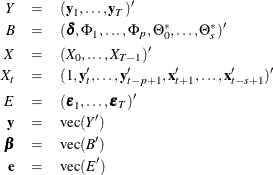The conditional least squares estimator of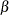can be obtained by using the same method in a VAR(p) modeling. If the multivariate linear model has different independent variables that correspond to dependent variables, the SUR (seemingly unrelated regression) method is used to improve the regression estimates.

The following example fits the ordinary regression model:

   proc varmax data=one;
model y1-y3 = x1-x5;
run;


This is equivalent to the REG procedure in the SAS/STAT software:

   proc reg data=one;
model y1 = x1-x5;
model y2 = x1-x5;
model y3 = x1-x5;
run;


The following example fits the second-order lagged regression model:

   proc varmax data=two;
model y1 y2 = x / xlag=2;
run;


This is equivalent to the REG procedure in the SAS/STAT software:

   data three;
set two;
xlag1 = lag1(x);
xlag2 = lag2(x);
run;

proc reg data=three;
model y1 = x xlag1 xlag2;
model y2 = x xlag1 xlag2;
run;


The following example fits the ordinary regression model with different regressors:

   proc varmax data=one;
model y1 = x1-x3, y2 = x2 x3;
run;


This is equivalent to the following SYSLIN procedure statements:

   proc syslin data=one vardef=df sur;
endogenous y1 y2;
model y1 = x1-x3;
model y2 = x2 x3;
run;


From the output in Figure 35.20 in the section Getting Started: VARMAX Procedure, you can see that the parameters, XL0_1_2, XL0_2_1, XL0_3_1, and XL0_3_2 associated with the exogenous variables, are not significant. The following example fits the VARX(1,0) model with different regressors:

proc varmax data=grunfeld;
model y1 = x1, y2 = x2, y3 / p=1 print=(estimates);
run;


Figure 35.45: Parameter Estimates for the VARX(1, 0) Model

The VARMAX Procedure

XLag
Lag Variable x1 x2
0 y1 1.83231 _
y2 _ 2.42110
y3 _ _

As you can see in Figure 35.45, the symbol ‘_’ in the elements of matrix corresponds to endogenous variables that do not take the denoted exogenous variables.